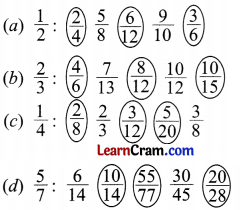# DAV Class 4 Maths Chapter 9 Worksheet 2 Solutions

The DAV Class 4 Maths Solutions and DAV Class 4 Maths Chapter 9 Worksheet 2 Solutions of Fractions offer comprehensive answers to textbook questions.

## DAV Class 4 Maths Ch 9 WS 2 Solutions

Question 1.
Encircle the equivalent fractions in each set of fractions.
(a) $$\frac{1}{2}: \frac{2}{4} \frac{5}{8} \frac{6}{12} \frac{9}{10} \frac{3}{6}$$
(b) $$\frac{2}{3}: \frac{4}{6} \frac{7}{13} \frac{8}{12} \frac{10}{12} \frac{10}{15}$$
(c) $$\frac{1}{4}: \frac{2}{8} \frac{2}{3} \frac{3}{12} \frac{5}{20} \frac{3}{8}$$
(d) $$\frac{5}{7}: \frac{6}{14} \frac{10}{14} \frac{55}{77} \frac{30}{45} \frac{20}{28}$$Question 2.
Tick (✓) the equivalent fractions.
(a) $$\frac{2}{3}, \frac{8}{12}$$ _________

(b) $$\frac{3}{4}, \frac{9}{16}$$ _________

(c) $$\frac{5}{6}, \frac{10}{18}$$ _________

(d) $$\frac{11}{12}, \frac{33}{36}$$ _________

(e) $$\frac{15}{16}, \frac{30}{36}$$ _________

(f) $$\frac{17}{20}, \frac{85}{100}$$ _________

Question 3.
Write the next four equivalent fractions for each.
(a) $$\frac{1}{3}, \frac{2}{6}, \frac{3}{9}$$, _____, _____, _____, _____
$$\frac{1}{3}, \frac{2}{6}, \frac{3}{9}, \frac{4}{12}, \frac{5}{15}, \frac{6}{18}, \frac{7}{21}$$

(b) $$\frac{2}{5}, \frac{4}{10}, \frac{6}{15}$$, _____, _____, _____, _____
$$\frac{2}{5}, \frac{4}{10}, \frac{6}{15}, \frac{8}{20}, \frac{10}{25}, \frac{12}{30}, \frac{14}{35}$$

(c) $$\frac{6}{10}, \frac{12}{20}, \frac{18}{30}$$, _____, _____, _____, _____
$$\frac{6}{10}, \frac{12}{20}, \frac{18}{30}, \frac{24}{40}, \frac{30}{50}, \frac{36}{60}, \frac{42}{70}$$

(d) $$\frac{3}{9}, \frac{6}{18}, \frac{9}{27}$$, _____, _____, _____, _____
$$\frac{3}{9}, \frac{6}{18}, \frac{9}{27}, \frac{12}{36}, \frac{15}{45}, \frac{18}{54}, \frac{21}{63}$$Question 4.
Write three equivalent fractions for each.
(a) $$\frac{1}{7}$$
$$\frac{2}{14}, \frac{3}{21}, \frac{4}{28}$$

(b) $$\frac{5}{8}$$
$$\frac{10}{16}, \frac{15}{24}, \frac{20}{32}$$

(c) $$\frac{9}{11}$$
$$\frac{18}{22}, \frac{27}{33}, \frac{36}{44}$$

(d) $$\frac{10}{13}$$
$$\frac{20}{26}, \frac{30}{39}, \frac{40}{52}$$

(e) $$\frac{8}{15}$$
$$\frac{16}{30}, \frac{24}{45}, \frac{32}{60}$$
(f) $$\frac{1}{25}$$
$$\frac{2}{50}, \frac{3}{75}, \frac{4}{100}$$
E.g. $$\frac{1}{3}=\frac{2}{6}, \frac{4}{12}$$
$$\frac{1^{\times 2}}{3_{\times 2}}=\frac{2^{\times 2}}{6_{\times 2}}=\frac{4}{12}$$